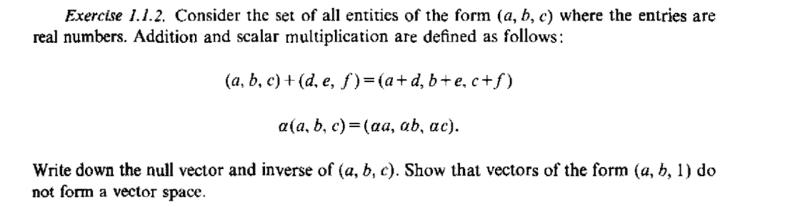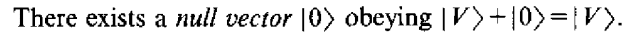# Show: Vectors e.g.(a,b,1) do not form vector space.

## Homework Statement## Homework Equations

definition of null vector,[/B]

## The Attempt at a Solution

null vector : ## |0 \rangle = (0,0,0) ##
inverse of (a,b,c) = ( - a, -b, -c)
vector sum of the two vectors of the same form e.g. (c,d,1) + ( e,f,1) = ( c+e, d+f, 2) does not have the same form. So, the vectors of the form ( a,b,1) do not form a vector space.

Is this correct?

#### Attachments

Last edited:

•